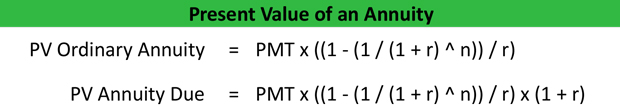# What is the Present Value of an Annuity?

Definition: The present value of an annuity is the amount of dollars today that a stream of equal future payments is worth. In other words, it’s the amount of money you would need to invest today in order to equate to the total of the annuity payments adjusted for the time value of money.

## What Does Present Value of an Annuity Mean?

What is the definition of present value annuity? An annuity is a financial instrument that provides regular payments to the holder each period until the end of the contract. The present value of these payments is the amount that an investor would have to invest today at a given interest rate to equate to the total amount of payments in the future discounted by the same interest rate.

Annuities are split into two main categorized: ordinary annuities and annuities due. In ordinary annuities, the payment is received at the end of the time period. Annuities due, on the other hand, receive their payments at the beginning of each period. The present value of an annuity formula is calculated below for both types of annuities.Where:
P = Present value of the annuity
PMT = Money value of each annuity payment R = discount rate
N = Number of periods / Number of payments made

Determining the present value of a stream of payments helps investors understand how much money they are actually receiving over time in today’s dollars and allows them to make informed investment decisions. This is a common calculation in most lottery winnings where the winner is usually offered the choice between being paid out a one-time lump sum or a series of payments over time. Most lottery winner typically choose the lump sum, so they can receive their winnings up front and invest them accordingly in the future.

Let’s look at an example.

## Example

Mr. Johnson is a 65 years old retired military veteran who has been funding his retirement account each month for the last 30 years and now he is finally able to start withdrawing funds. As part of the agreement, the retirement company is offering to pay him \$30,000 the 1st of each year for the next 25 years, or a one-time payment of \$500,000. He wants to know what the value of the \$30,000 yearly payments is worth today to determine his best option.

Using the present value formula above, we can see that the annuity payments are worth about \$400,000 today assuming an average interest rate of 6 percent. Thus, Mr. Johnson is better off taking the lump sum amount today and investing it himself.

## Summary Definition

Define Present Value of an Annuity: PV of an Annuity means the dollar amount a stream of equal payments in the future is worth today.